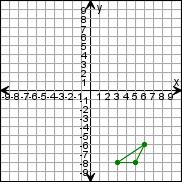Create a new printableGeometry
Math Worksheets

Sample - Click above to make a new math worksheet (PDF).
 Name _____________________________Date ___________________
Transformations
Draw the triangle after the transformations.

 1 Rotate the triangle 270clockwise around (0,0).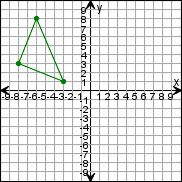2 Rotate the triangle 180clockwise around (0,0).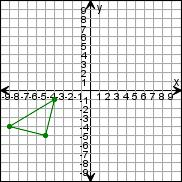3 Rotate the triangle 180clockwise around (0,0).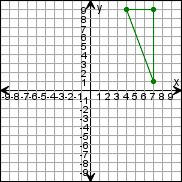4 Rotate the triangle 90clockwise around (0,0).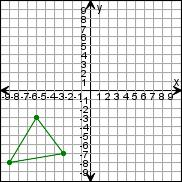5 * This is a pre-made sheet.Use the link at the top of the page for a printable page.
 6 Rotate the triangle 270clockwise around (0,0).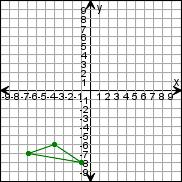7 Rotate the triangle 180clockwise around (0,0).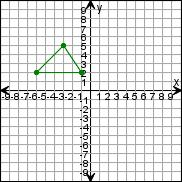8 Rotate the triangle 180clockwise around (0,0).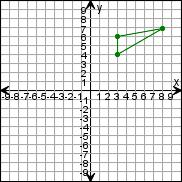9 Rotate the triangle 90clockwise around (0,0).